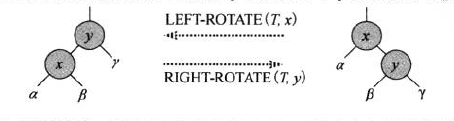# 算导学习——红黑树

## 定义

1. 每个结点要么红色，要么黑色
2. 根节点黑色
3. 每个页结点(NIL)黑色
4. 如果一个节点红色，则其两个子结点黑
5. 对每个结点，从该节点到其所有后代叶结点的简单路径上，均包含相同数目的黑色结点。

## 性质

• 任一结点x为根的子树中，至少包含$$2^{bh(x)} -1$$个内部结点

$$\text{set the number of inner node is }inner\_node$$

$$\text{if the height of x is 0, it means x is the nil, and } bh(x) = 1$$

$$\text{so } inner\_node \ge 2^{bh(x)} - 1 = 0.\ \ \text{satisfaction}$$

$$\text{when the height of x is not 0, the black height of its sub tree is } bh(x) \text{ or } bh(x) - 1$$

$$so, inner\_node\_single\_sub\_tree \ge 2^{bh(x)} - 1$$

$$then, inner\_node \ge (2^{bh(x)-1} - 1) + (2^{bh(x)-1} - 1) + 1 = 2^{bh(x)} - 1$$

• 一颗有n个内部节点的红黑树高度至多为$$2log(n+1)$$

## 相关操作

### 树的旋转删除

• $$\alpha$$：减一
• $$\beta$$：不变
• $$\gamma$$：加一

### 插入

2.根节点黑色

4.如果一个节点红色，则其两个子结点黑

5.对每个结点，从该节点到其所有后代叶结点的简单路径上，均包含相同数目的黑色结点。

• 插入红结点有更小的可能性改变红黑性质

插入黑结点必然会改变性质5，使不同路径的黑高不同。但是插入红结点不会改变树的黑高。当树非空时，只会有一半左右的几率破坏性质4

• 插入红结点破坏的性质更容易修复

相较于修复不同路径黑高的不同，修复对性质4——红结点的后代只能为黑结点——的破坏更为容易。

#### 修复可能被破坏的红黑性质

rbInsertFixUp(rbTree T, node z)中，在执行每次操作前，保证如下3个部分不变式：

• 节点z是红结点

• 如果z->parent是根结点，则z->parent是黑结点

• 如果有任何红黑性质的破坏，则至多只有一条被破坏，或是性质2,或是性质4.

如果被破坏的是性质2, 则原因只能为z是根节点且是红结点。

如果被破坏的是性质4,则原因只能是z和z->parent均为红结点。

• 情况1： z的叔结点y是红色的

• 情况2: z的叔结点y是黑色的且z是一个右孩子
• 情况3: z的叔结点y是黑色的且z是一个左孩子

#### 复杂度分析

rbInsert的总时间复杂度为$$O(log(n))$$

### 删除

• 如果z的子结点全为NIL，则直接删除z即可
• 如果z仅有一个子结点，则删除z，并让z的非NIL子结点取代z的位置即可
• 如果z有两个子结点，考虑到要维持BST的有序性，则必须取z的后继(BST中比z大的第一个数)y，使其代替z的位置，并将z的左右子树分别作为该后继的左右子树。

• z至多只有一个子结点

当z的颜色为黑时，会破坏黑高

• z有两个子结点

当y的颜色为黑时，会破坏黑高

• 如果y是根结点，则破坏了性质2——根结点必须为黑色。
• 如果x和x.parent均为红色，则破坏了性质4——如果一个节点红色，则其两个子结点黑。
• 删除y使得任一包含y的简单路径上的黑高-1，因此y的任何一个祖先都不满足性质5——黑高相等。

y原来的位置如今由x代替。在x上额外加上一层黑色，以此代替被删除的结点y。如此一来性质5便没有被破坏。但是此时结点x的颜色就变成了红黑色或者黑黑色，违背了性质1——结点颜色要么黑，要么红。

• case 1: x的兄弟结点w是红色的

• case 2: x的兄弟结点w是黑色的，且w的两个子结点都是黑色的

• case 3: x的兄弟结点w是黑色的，且w的左孩子是红色的，w的右孩子是黑色的

• case 4: x的兄弟结点w是黑色的，且w的右孩子是红色的

## 总结

《算法导论》

stack overflow: Why are newly inserted nodes are always colored red in red black tree insert operation?